Please Note: This article is written for users of the following Microsoft Excel versions: 2007, 2010, 2013, 2016, 2019, Excel in Microsoft 365, and 2021. If you are using an earlier version (Excel 2003 or earlier), this tip may not work for you. For a version of this tip written specifically for earlier versions of Excel, click here: Decimal Tab Alignment.

# Decimal Tab AlignmentWritten by Allen Wyatt (last updated September 17, 2022)
This tip applies to Excel 2007, 2010, 2013, 2016, 2019, Excel in Microsoft 365, and 2021

If you have ever aligned numeric information in Word using decimal tabs, you know they can be very handy. The tabs even align text (with no decimal point) to the left of an assumed decimal point, with everything nice and tidy.

Unfortunately, Excel has no such similar feature as a "decimal tab." While it is very easy to get things lined up if they include decimals (at least if they contain the same number of digits to the right of the decimal), adding text into a cell can throw everything out of whack.

To closely approximate the behavior of decimal tab alignment, follow these steps:

1. Select the cells you want to format.
2. Display the Home tab of the ribbon.
3. Click the small icon at the bottom-right of the Number group. Excel displays the Format Cells dialog box.
4. Make sure the Number tab is selected. (See Figure 1.)
5.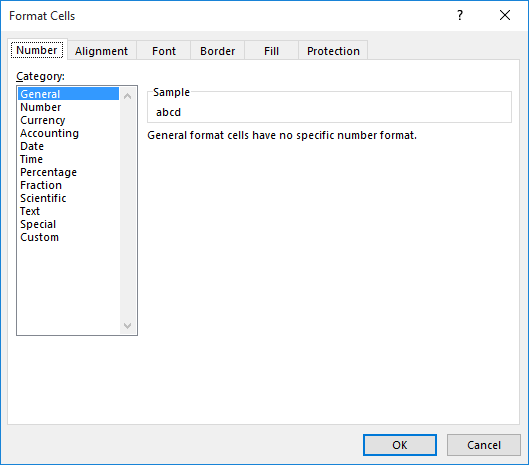Figure 1. The Number tab of the Format Cells dialog box.

6. In the Category list, choose Custom.
7. In the Type box, enter the following format:
8. ```         _(* #,##0.00_);_(* (#,##0.00);_(* "-"??_);_(@_._0_0_)
```
9. Display the Alignment tab of the dialog box. (See Figure 2.)
10.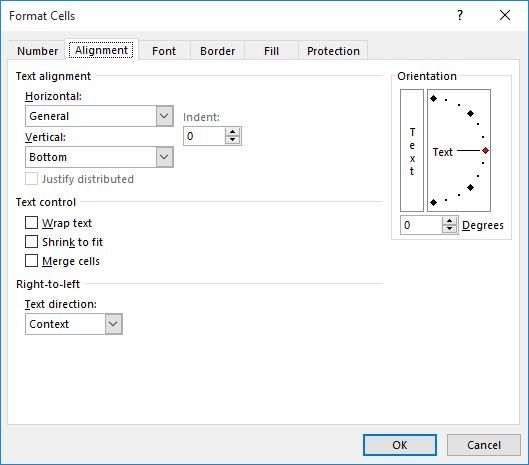Figure 2. The Alignment tab of the Format Cells dialog box.

11. Using the Horizontal drop-down list, choose Right.
12. Click on OK.

The format you are setting up in step 6 allows for two decimal places and parentheses around negative numbers. In addition, it leaves room after the text for a period, two zeros, and the optional closing bracket. Step 8 is necessary so that Excel pushes text up to the right end of the cell. Since the format you specified leaves room for the decimal point and everything after it, the text appears to align just to the left of where the period would appear.

Understand that this is only an approximation of the decimal tab alignment offered in Word. There are still a few things you can't do. In Word, if you enter text and it is decimal aligned, and the text includes a period, then the period is aligned as if it were a decimal point. If you put a period in the text entered in a cell that is formatted as directed above, the period will not be treated as a decimal point.

ExcelTips is your source for cost-effective Microsoft Excel training. This tip (12318) applies to Microsoft Excel 2007, 2010, 2013, 2016, 2019, Excel in Microsoft 365, and 2021. You can find a version of this tip for the older menu interface of Excel here: Decimal Tab Alignment.

##### Author Bio

Allen Wyatt

With more than 50 non-fiction books and numerous magazine articles to his credit, Allen Wyatt is an internationally recognized author. He is president of Sharon Parq Associates, a computer and publishing services company. ...

##### MORE FROM ALLEN

Creating a Footer

Adding a predefined footer to your worksheets is easy, and it helps convey valuable information when you make a printout. ...

Discover More

Setting the Return Address Used in Word

When you create envelopes with Word, it normally displays a return address by default. If you can't get Word to retain ...

Discover More

Mail Merge and Data Source Documents become Unattached

When you create a mail merge document, you attach it to a data source that is the basis for the information to be merged ...

Discover MoreCreate Custom Apps with VBA! Discover how to extend the capabilities of Office 2013 (Word, Excel, PowerPoint, Outlook, and Access) with VBA programming, using it for writing macros, automating Office applications, and creating custom applications. Check out Mastering VBA for Office 2013 today!

##### More ExcelTips (ribbon)

Saving Custom Formats

While the implementation of custom formats in Excel is not terribly robust, you can still achieve some amazing results ...

Discover More

Notation for Thousands and Millions

When working with very large numbers in a worksheet, you may want the numbers to appear in a shortened notation, with an ...

Discover More

Getting Rid of Leading Zeros in a Number Format

Excel, by default, displays numbers with a leading zero, if they are less than 1. Here's how you can get rid of those ...

Discover More
##### Subscribe

FREE SERVICE: Get tips like this every week in ExcelTips, a free productivity newsletter. Enter your address and click "Subscribe."

If you would like to add an image to your comment (not an avatar, but an image to help in making the point of your comment), include the characters [{fig}] (all 7 characters, in the sequence shown) in your comment text. You’ll be prompted to upload your image when you submit the comment. Maximum image size is 6Mpixels. Images larger than 600px wide or 1000px tall will be reduced. Up to three images may be included in a comment. All images are subject to review. Commenting privileges may be curtailed if inappropriate images are posted.

What is 9 + 4?

2023-01-05 17:08:35

Tomek

Thank you Allen for cleaning up my failed postings.

If anyone has problems opening the .bas file I mentioned in my earlier comment (after all, it contains the code that could be malicious), I posted the code also as a .txt file

https://1drv.ms/t/s!AnwQtlPv1u28gvg1R2zvOwCHM2kLKA?e=2wDrIk

2023-01-05 00:20:35

Tomek

Well, I give up. The website still collapsed the search text from "  " to " " i.e., two hard spaces to a single space.
It should be in the first search and replace block:
With Selection.Find
.Text = "  " 'two spaces between quotation marks.

So, I have posted the code in my OneDrive as a PrepCodeforPosting.bas file you can access by the following link
https://1drv.ms/u/s!AnwQtlPv1u28gvgxXRaJv0Xorr1aBA?e=8ccq0C

This should work without formatting problems. Please ignore any earlier code I posted in these comments.

Allen, I apologize for cluttering comments to this tip, I did not realize how many traps I will fall into. I hope you can clean up (remove) my earlier postings (the ones with the macro code).

2023-01-04 11:32:21

Tomek

As for posting formatted (indented) code I find it much easier to follow than the plain text. So I would strongly encourage everyone to do this, although it requires extra effort. As James pointed out this was discussed in the past. At one point I collaborated with @Kiwerry who created a MS Word macro based on my idea of using nonbreakable space HTML code (&​nbsp;) to replace regular spaces. It yields an ugly-looking text, which when posted in these Tips comments does show up with proper indentations, but what is more important, it can be copied and pasted ***directly*** into the VBA editor. All non-breakable spaces are converted automatically back to regular ones leaving nicely indented code.

I will post the macro code in a separate comment with Kiwerry's permission to do it.

2023-01-04 00:29:09

Tomek

@Peter and J. Woolley,
Thank you for your replies. I was not using a computer for a few days, and couldn't do testing of your macros yet, but I am planning to do it soon.

2023-01-02 15:36:42

J. Woolley

My Excel Toolbox includes the following function to return a numeric Value (text or number) and align its decimal point at DecimalDigits without insignificant trailing zeroes:
=DecimalAlign(Value,DecimalDigits,[BaseFormat],[NegParen],[NegRed])
Value can be a constant, a formula, or a cell reference like \$A\$1; if blank, alphabetic, or logical it will not be altered.
BaseFormat must not include a period or semicolon (like "#,##0"); default is "0".
If NegParen is TRUE, Value will have surrounding parentheses if negative or matching space if positive. If NegRed is TRUE, Value will have red font if negative. If both NegParen and NegRed are FALSE (default), Value will be preceded by minus if negative.

2022-12-29 11:49:08

J. Woolley

When comments are posted to Tips.Net, the resulting HTML apparently trims all spaces except single spaces between words. This removes leading space characters from indented VBA code, which makes it difficult to read.
Before posting VBA code in a comment, I copy it (Ctrl+C) from Excel's VB Editor (Alt+F11) and paste it (Ctrl+V) into Notepad. Then I use Ctrl+H in Notepad to replace leading space characters with non-breaking space characters (Alt+0160, numeric keypad) in groups of four. Finally I copy my comment from Notepad (Ctrl+C) and use the Chrome browser to paste it into Tips.Net (Ctrl+V).
Later when I view that Tips.Net comment in Chrome, if I select the VBA code with my mouse and copy it (Ctrl+C) then paste it (Ctrl+V) into a module's code pane in Excel's VB Editor, the leading non-breaking space characters are converted back to regular space characters. I use Excel 365, so I don't know if earlier versions of the VB Editor behave the same. If not, simply select the first four non-breaking space characters in VB Editor, then press Ctrl+H to replace all non-breaking space characters with regular space characters in groups of four.
Here are some suggestions I try to remember before posting comments with VBA code to Tips.Net:
1. Carefully test the code first.
2. Limit line length to 80 characters.
3. Minimize comments within the code.
4. Avoid blank lines.
5. Wait until tomorrow because something will change.
6. If the code is too long or complicated, it can always be shared in the cloud (like Google Drive) with a link posted to Tips.Net.
See David Bonin, 2021-01-18, https://excelribbon.tips.net/T011598
See Tomek, 2021-11-08, https://excelribbon.tips.net/T011193
See Tomek, 2021-11-19, https://excelribbon.tips.net/T012611

2022-12-27 10:16:15

J. Woolley

@Petter
You misspell my name, too.
I use Chrome. If I select the VBA code from my comment below and press Ctrl+C to copy, then open the VB Editor and press Ctrl+V to paste into a module's code pane, it is aligned perfectly. Do not paste into a text editor like Notepad or Word first. How did you copy-paste the code?

2022-12-27 05:51:30

Peter Atherton

J Wooley
I tried running your code but it did not work. Copying it into Word I see that it is littered with non-breaking spalces and soft returns that the VBA editor can't interperate. Perhaps it might be better if you post the plain text. In the mean time here's my editted code.
'=====================
Option Explicit
Dim r As Range, c As Range, ndp As Integer

Sub Align2Dec2()
'Allen Wyatt
'https://excelribbon.tips.net/T012318_Decimal_Tab_Alignment.html
Dim dp As Integer, sSpacer As String, sNumber As String, _
n As Integer
Dim showIntDec As Boolean
'showIntDec = True
Const NUMBERFMT As String = "#,##0."
sSpacer = "_#"
sNumber = "#"

'This is a test variable to help decide the way to display the formatted data. _
suggest commenting it out and change the variable above o true or valse when _
you have decided
showIntDec = Application.InputBox("To Show Decimal point enter 1" & vbLf _
& "To Hide Decimal Point enter 0", "Integer Decimal Show/Hide", False, Type:=4)

Set r = Selection
GetNDP2a

For Each c In r
dp = 0
On Error Resume Next
With Application.WorksheetFunction
dp = Len(c) - .Find(".", c)
n = ndp - dp
'If n > 9 Then n = 9
If dp = 0 Then
'don't show decimal
If Not showIntDec Then
c.NumberFormat = "#,##0" & .Rept(sSpacer, ndp + 1)
Else
c.NumberFormat = NUMBERFMT & .Rept(sSpacer, ndp)
End If
Else
c.NumberFormat = NUMBERFMT & .Rept(sNumber, dp) & .Rept(sSpacer, n)
End If
End With
c.Formula = c.Formula
Next c
End Sub

'Get number of decimal places
Private Sub GetNDP2a()
Dim dp As Integer
'Set r = Selection
ndp = 0
'Get max Number of Decimal places
For Each c In r
With Application.WorksheetFunction
On Error Resume Next
dp = Len(c) - .Find(".", c)
If IsError(dp) Then dp = 0
If dp > ndp Then
ndp = dp
End If
End With
Next
End Sub

'Reset selection format
Sub ResetFormat()
Selection.NumberFormat = "general"
End Sub

(see Figure 1 below)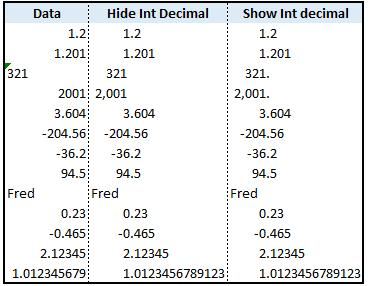Figure 1.

2022-12-26 12:31:50

Peter Atherton

J Wooley

f (see Figure 1 below)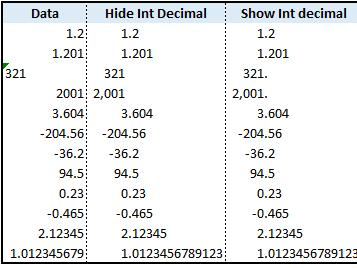Figure 1.

2022-12-26 12:05:17

J. Woolley

@Tomek
I hope this macro will satisfy your requirements. It inserts a new adjacent column with the results, so the original source is not disturbed. (see Figure 1 below) Numeric values are copied as numbers, not text, with custom format; other values (alphabetic, logical, date) are copied directly. The following numeric formats are NOT preserved: Currency, Accounting, Time, Percentage, Fraction, Scientific, Special, Custom. Since formulas are copied as formulas, recalculation might misalign a formula's result.
This macro could be modified to align decimal formats insitu similar to Peter's macro.

Sub AlignDecimal()
Dim msg As String, rSele As Range
Dim nBeg As Long, nEnd As Long, nUsed As Long, nCells As Long
Dim nDec As Integer, maxDec As Integer, nDecDigits() As Integer
Dim sVal As String, sFmt As String, i As Integer, n As Long
Const myName As String = "AlignDecimal"
Const limDec As Integer = 9 'default is 15
Const baseFmt As String = "0" 'or perhaps "#,##0"
msg = "Use the mouse to select a column vector range of cells " & _
"needing decimal alignment. A new adjacent column will be " & _
"inserted with the result."
Set rSele = Nothing
On Error Resume Next
Set rSele = Application.InputBox(msg, myName, sVal, Type:=8)
On Error GoTo 0
If rSele Is Nothing Then Exit Sub
Set rSele = rSele.Columns(1)
With rSele
nBeg = .Row
nEnd = nBeg + .Rows.Count - 1
n = .Column
nUsed = Columns(n).Find(What:="*", After:=Columns(n).Cells(1), _
LookAt:=xlPart, LookIn:=xlFormulas, SearchOrder:=xlByRows, _
SearchDirection:=xlPrevious, MatchCase:=False).Row
End With
If nEnd > nUsed Then Set rSele = rSele.Resize(nUsed - nBeg + 1)
With rSele
nCells = .Cells.Count
ReDim nDecDigits(1 To nCells)
For n = 1 To nCells
sVal = CStr(.Cells(n).Value)
If IsNumeric(sVal) Then
nDec = Len(sVal) - InStr(1, sVal, ".")
If nDec = Len(sVal) Then nDec = 0
If nDec > limDec Then nDec = limDec
If maxDec < nDec Then maxDec = nDec
Else
nDec = -1
End If
nDecDigits(n) = nDec
Next n
.EntireColumn.Offset(0, 1).Insert
For n = 1 To nCells
nDec = nDecDigits(n)
If nDec > -1 Then
If nDec = 0 Then
sFmt = "_."
Else
sFmt = "." & String(nDec, "0")
End If
For i = (nDec + 1) To maxDec
sFmt = sFmt & "_0"
Next i
.Cells(n).Offset(0, 1).NumberFormat = baseFmt & sFmt
End If
.Cells(n).Offset(0, 1).Formula = .Cells(n).Formula
Next n
End With
End Sub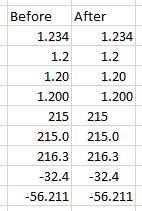Figure 1.

2022-12-26 11:00:02

J. Woolley

@Peter
I was working on my own macro; see my next comment.
Your macro doesn't work if the numeric value was actually text; to fix it, add this statement immediately before 'Next c':
c.Formula = c.Formula
Also, I was wrong about the hash (#) characters after the decimal point (.) in my previous comment; they should be zero (0) characters. Change your macro as follows:
sSpacer = "_0" 'not "_#"
sNumber = "0" 'not "#"

2022-12-26 06:01:31

Peter Atherton

Based on J Wooley's ecellent suggestion, there is no need to hard code the formats and just set the format on the fly.

Option Explicit
Dim r As Range, c As Range, ndp As Integer

Sub Align2Dec2()
'Based on idea by J Wooley, 23 Dec 2022
'https://excelribbon.tips.net/T012318_Decimal_Tab_Alignment.html
Dim dp As Integer, sSpacer As String, sNumber As String, _
n As Integer
Dim showIntDec As Boolean
'showIntDec = True
Const NUMBERFMT As String = "#,##0."
sSpacer = "_#"
sNumber = "#"

'This is a test variable to help decide the way to display the formatted data. _
suggest commenting it out and change the variable above o true or valse when _
you have decided
showIntDec = Application.InputBox("To Show Decimal point enter 1" & vbLf _
& "To Hide Decimal Point enter 0", "Integer Decimal Show/Hide", False, Type:=4)

Set r = Selection
GetNDP2a

For Each c In r
dp = 0
On Error Resume Next
With Application.WorksheetFunction
dp = Len(c) - .Find(".", c)
n = ndp - dp
'If n > 9 Then n = 9
If dp = 0 Then
'don't show decimal
If Not showIntDec Then
c.NUMBERFORMAT = "#,##0" & .Rept(sSpacer, ndp + 1)
Else
c.NUMBERFORMAT = NUMBERFMT & .Rept(sSpacer, ndp)
End If
Else
c.NUMBERFORMAT = NUMBERFMT & .Rept(sNumber, dp) & .Rept(sSpacer, n)
End If
End With
Next c
End Sub
Sub GetNDP2a()
Dim dp As Integer
'Set r = Selection
ndp = 0
'Get max Number of Decimal places
For Each c In r
With Application.WorksheetFunction
On Error Resume Next
dp = Len(c) - .Find(".", c)
If IsError(dp) Then dp = 0
If dp > ndp Then
ndp = dp
End If
End With
Next
End Sub

I tested this with an 11 dp number.

2022-12-23 10:35:34

J. Woolley

@Tomek
These custom formats will align the decimal when there are N significant digits after the decimal point:
If N=3, use #,##0.###
If N=2, use #,##0.##_#
If N=1, use #,##0.#_#_#
If N=0, to keep the decimal use #,##0._#_#_#
If N=0, to hide the decimal use #,##0_._#_#_#

2022-12-23 07:25:56

Peter Atherton

Tomek, This is my Test data

(see Figure 1 below)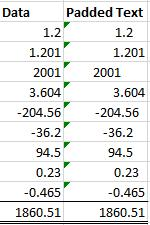Figure 1.

2022-12-23 07:19:36

Peter Atherton

Tomek
This converts everything to a string. This will need an array formula to say add up.
The variable ndp is declared at the top of the module and is used by both routines.

Dim ndp As Integer
Dim r As Range, c As Range, dp As Integer
Dim spacer As String, nspacers As Integer

spacer = " "
Set r = Selection
'get max decimal points
GetNDP
For Each c In r
With WorksheetFunction
'How many Decimals
On Error Resume Next
dp = Len(c) - .Find(".", c)
nspacers = ndp - dp
If dp = ndp Then
c = "'" & c
ElseIf dp = 0 Then
'Extra space for decimal dot
c = "'" & c & .Rept(spacer, nspacers + 1)
Else
c = "'" & c & .Rept(spacer, nspacers)
End If
End With
dp = 0
Next
r.HorizontalAlignment = xlRight
End Sub
Sub GetNDP()
Dim r As Range, c As Range, dp As Integer
Set r = Selection
ndp = 0
'Get max Number of Decimal places
For Each c In r
With Application.WorksheetFunction
On Error Resume Next
dp = Len(c) - .Find(".", c)
If IsError(dp) Then dp = 0
If dp > ndp Then
ndp = dp
End If
End With
Next

End Sub

Hope this helps

2022-12-22 23:09:33

Tomek

Peter and Steve,
Thank you for your responses. Your macros both do the decimal alignment, but also they modify the number of displayed significant digits by padding the number with trailing zeroes. What I wanted to achieve is alignment without rounding or padding the number with zeroes. Rather I would like padding to be with spaces, but significant trailing zeroes should be kept. I can do it manually for each number, but not by formatting or even macro.

To see what I mean (see Figure 1 below) . I run your macros on a sample data and the results are shown.

For my purposes, I don't need brackets/red for negatives, nor aligning of the text.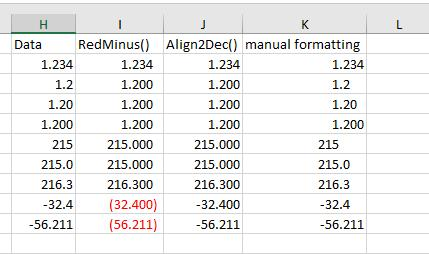Figure 1. Exampe of Alignments

2022-12-22 12:12:01

Tomek

Peter and Steve,
Thank you for your responses. Your macros both do the decimal alignment, but also they modify the number of displayed significant digits by padding the number with trailing zeroes. What I wanted to achieve is alignment without rounding or padding the number with zeroes. Rather I would like padding to be with spaces, but significant trailing zeroes should be kept. I can do it manually for each number, but not by formatting or even macro.

To see what I mean (see Figure 1 below) . I run your macros on a sample data and the results are shown.

For my purposes, I don't need brackets/red for negatives, nor aligning of the text.Figure 1. Exampe of Alignments

2022-12-22 09:07:51

Peter

Oops, the lat line should have been
r.NumberFormat = "0." & WorksheetFunction.Rept(0, ndp)

Don't know how I did that

2022-12-22 07:13:40

Peter Atherton

This macro works, just changes format

Sub Align2Dec()
Dim r As Range, c As Range, ndp As Integer, dp As Integer
Dim nf As String
Set r = Selection

ndp = 0
'Get max Number of Decimal places
For Each c In r
With Application.WorksheetFunction
On Error Resume Next
dp = Len(c) - .Find(".", c)
If IsError(dp) Then dp = 0
If dp > ndp Then
ndp = dp
End If
End With
Next
r.NumberFormat = "0." & WorksheetFunction.Rept(2, ndp)

End Sub

2022-12-21 07:32:53

Steve J

Posted the last one to quickly.
The code runs through the selection, finds the largest number of decimal places & uses that to format the number of decimal places for the range.

It doesn't deal with text yet, but that would be possible to add.

S

2022-12-21 07:29:00

Steve J

@ Tomek

The following will generate output as fig 1. not sure if that's what you need.
You just need to select a range & run the code.

Sub RedMinus()

Dim rng
Dim dl As Integer, ds As Integer
Dim dp As String

rng = Selection
ds = 0

For Each c In rng
If c = "" Then
GoTo nxt

Else
On Error Resume Next
dl = Application.WorksheetFunction.Max(Application.WorksheetFunction.IfError(Len(c), 0) - Application.WorksheetFunction.IfError(Application.WorksheetFunction.Find(".", c), 0))

If dl > ds Then
ds = dl

End If
End If
nxt:

Next c

dp = Application.WorksheetFunction.Rept(0, ds)

Selection.NumberFormat = "#,##0." & dp & "_) ;[Red](#,##0." & dp & ")_ ;;@"

End Sub

HTH I'm pretty sure there is much more efficient / elegant way of doing this, but hey it works...

(see Figure 1 below)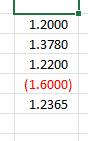Figure 1. Fig 1

2022-12-21 02:33:17

Tomek

larrybud:
it allows for proper alignment of positive numbers, negative numbers (displayed in parentheses), zero, and even text right-aligned with the decimal point in numbers in other cells.
Formatting to just two decimal places does not allow negative numbers shown in parentheses to align properly, nor zeroes nor text.

Having said that, it still is not a solution to decimal align numbers with varying number of significant digits to the right of decimal point, for example numbers 1.27, 1.345, 1.200, 1.2, and 1270 (without decimal point) will not align properly or will be rounded. Note that in science 1.2 and 1.200 although seemingly are the same value, they are not, the latter implies the accuracy of +/- 0.0005, the former of +/-0.05.
I was never able to successfully create such alignment in Excel or Access, and also had problems with keeping the trailing significant zeroes , so once the data was in the table I resorted to programatically creating a report as a Word document, where the alignment could be easily achieved.
If someone has a solution for this, please let me know.

2022-12-19 20:02:02

larrybud

How is this any different than using the number format to 2 decimal places?

##### This Site

Got a version of Excel that uses the ribbon interface (Excel 2007 or later)? This site is for you! If you use an earlier version of Excel, visit our ExcelTips site focusing on the menu interface.